# C++ Program to Display Factors of a Number

In this tutorial you will learn about the C++ Program to Display Factors of a Number and its application with practical example.

In this tutorial, we will learn to create a C++ program that will find Factors of a number using C++ programming.

## Prerequisites.

Before starting with this tutorial we assume that you are best aware of the following C ++ programming topics:

• Operators.
• If else statement.
• For Loop.
• Basic input/output.
• Basic c++ Programming.

## What Is Factor of number is?

Factors of a number are numbers that divide the number completely and remainder of that number(Divisor) is equal to zero .

## Example:->

The number 6 has four factors: 1, 2, 3,  and 6 because all this number divide the number six completely.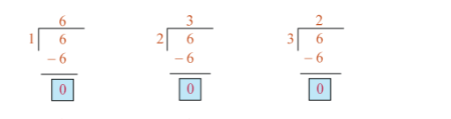## C++ Program to Display Factors of a Number

In this program we find factor of given number using if -else statement and for loop. First we would declared and initialized the required variables. Next, we would prompt user to input the number . Later we will find factors of a given number.

## Output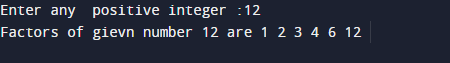In this program  we will take a positive integer from user by prompting user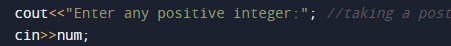and with the help of loop and if else statement  we will displays all the positive factors of that number.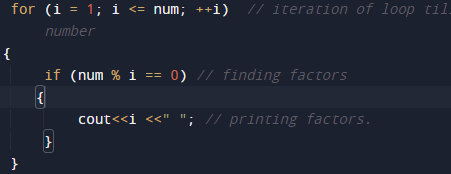In the above program, we have first declared and initialized a set variables required in the program.

• num = It will hols number given by user.
• = for iteration of loop and finding  factor.

So finding the factors of a number  we will run a loop from 1 to num, incrementing  the loop value by 1 in each iteration.

The loop structure should look like thisWithin the loop  in each iteration we check the condition that i is a factor of num or not. To check factor we use divisibility of number by using  modulo division.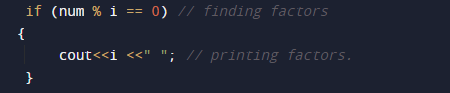i.e. if( num % i == 0) then i is a factor of num If i is a factor of num then we will print the value of i.So we will get the required factors of given number.In this tutorial we have learn about the C++ Program to Display Factors of a Number and its application with practical example. I hope you will like this tutorial.